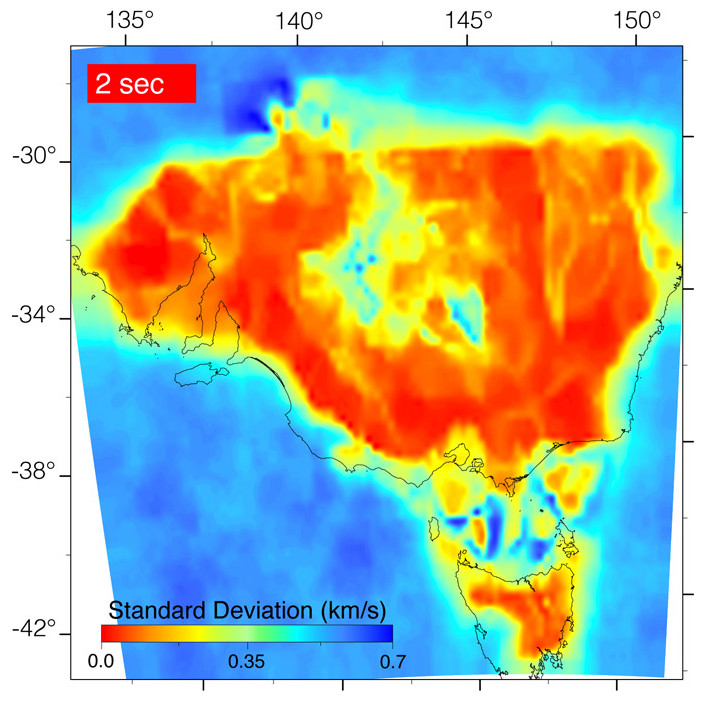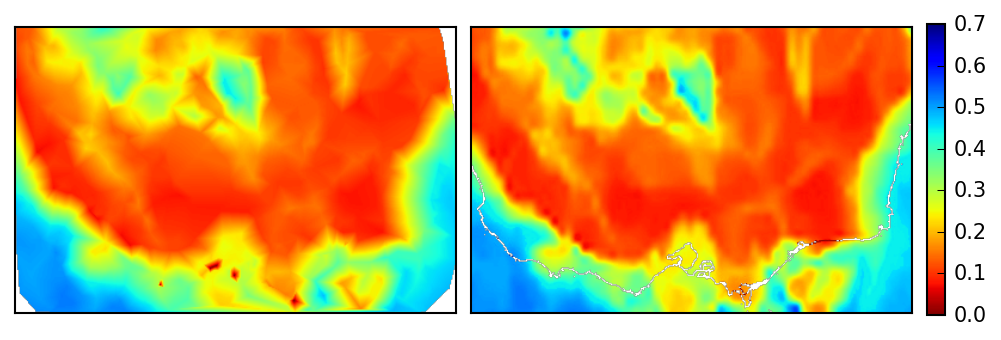# Convert images to arraysConvert any image to data array

Oftentimes, you will have an image that you want to extract data from. It will most likely be something annoyingly minor, but will somehow turn into the most important piece to the puzzle that you NEED RIGHT NOW! Okay… maybe that’s just me. Sometimes it’s nice to be able to extract data from an image you found in a journal article to replicate the results.

Here, I will go through the steps I took to convert an image into RGB format (using Python - naturally) and convert it into an array of data. From there it gets interesting. A lot of images I come across have been interpolated from relatively sparse data. You don’t need all that data! It’s a waste of space and you’re better off trimming the bits you don’t actually need - especially if you would like to do some spatial post-processing.

Here are the main steps:

1. Import the image using the scipy.ndimage module.
2. Setup a colour map using matplotlib.colors
3. Query the colour map with many numbers along the interval you’ve defined and store that in a KDTree.
4. Query the KDTree using the $m \times n \times 3$ ndimage RGB array. The result is an $m \times n$ data array.
5. Reduce the data by taking the first spatial derivative using numpy.diff and removing points where $|\nabla| > 0$.
6. Use sklearn.DBScan from the scikit-learn module to cluster the data and remove even more unnecessary data.
7. Have a beer.

At the end I have a Python class that contains all these steps rolled into one nifty code chunk for you to use on your own projects, if you find this useful.

from scipy import ndimage
import numpy as np
import matplotlib.pyplot as plt

im = ndimage.read("filename.jpg") # flatten=True for b&w
plt.imshow(im)
plt.show()That looks nice, im is a $m \times n \times 3$ array - i.e. 3 sets of $m$ rows, $n$ columns, that correspond to the number of pixels, for each RGB channel. Now let’s create the colour map and chuck everything into a KDTree for nearest-neighbour lookup. Seriously, KDTrees are awesome. srsly.

from scipy.spatial import cKDTree
from matplotlib import colors as mcolors

def scalarmap(cmap, vmin, vmax):
""" Create a matplotlib scalarmap object from colour stretch """
cNorm = mcolors.Normalize(vmin=vmin, vmax=vmax)
return plt.cm.ScalarMappable(norm=cNorm, cmap=cmap)

scalarMap = scalarmap(plt.get_cmap("jet_r"), 0.0, 0.7)
value_array = np.linspace(0,0.7,10000)
color_array = np.asarray(scalarMap.to_rgba(value_array))
color_array *= 255 # rescale

tree = cKDTree(color_array[:,:-1]) # Last column is alpha
d, index = tree.query(im)
data = value_array[index]


We scaled the colour map between 0.0 and 0.7, which corresponds to the extent in the image. Optionally, we could mask pixels that are some distance, $d$, from the colour map. E.g. data = np.ma.array(value_array[index], mask=(d > 70)). This would mask out the black text and white background in the image.

I don’t know a better way to map the RGB colour arrays to data than to query entries in a KDTree. If you do, please mention in the comments below.

## Data reduction

Now that we have converted an image into an array we can reduce the data so that only the important bits remain. Firstly, we take the first spatial derivative and find where $\nabla = 0$.

dx = np.abs(np.diff(data[:,:-1], axis=0))
dy = np.abs(np.diff(data[:-1,:], axis=1))
dxdy = (dx + dy)/2.0



Here, mask is a $m \times n$ boolean array that contains True values where we have good data. But we can reduce it even further using DBScan. This finds spatial regions of high density and expands clusters from them. I think this is basically a higher order use of KDTrees, but let’s humour sklearn toolkit anyway.

from scikit.cluster import DBSCAN

Xcoords = np.arange(data.shape-1)
Ycoords = np.arange(data.shape-1)
xx, yy = np.meshgrid(Xcoords, Ycoords)

labels = db.labels_
num_clusters = len(set(db.labels)) - (1 if -1 in db.labels else 0)

def get_centroid(points):
""" find centroid of a cluster in xy space """
n = points.shape
sum_lon = np.sum(points[:, 0])
sum_lat = np.sum(points[:, 1])
return (sum_lon/n, sum_lat/n)

x = np.zeros(num_clusters, dtype=int)
y = np.zeros(num_clusters, dtype=int)

for i in xrange(num_clusters):

centroid = get_centroid(np.vstack([xc,yc]).T)

d, index = tree.query(centroid)
x[i], y[i] = tree.data[index]



Above, we found the centroids for each cluster to reduce the substantially reduce the dataset from 16667 points down to 1221. Each colour represents a unique cluster in the image below.mask2 has significantly improved on mask by finding clusters in the data. Now that it’s done, let’s see if we can reconstruct the image we first started with. This is a good check to make sure we haven’t removed too much of the original data.Overall the two look pretty similar! There are some bits that could be refined by adjusting some of the parameters, e.g. eps in DBScan and $\nabla \approx 0$. Also, adjusting the distance threshold in the original RGB $\rightarrow$ data conversion would remove artifacts caused by the coastline (i.e. bits that don’t find on the colour map).##### Dr. Ben Mather
###### Computational Geophysicist

My research interests include Bayesian inversion, Python programming and Geodynamics.